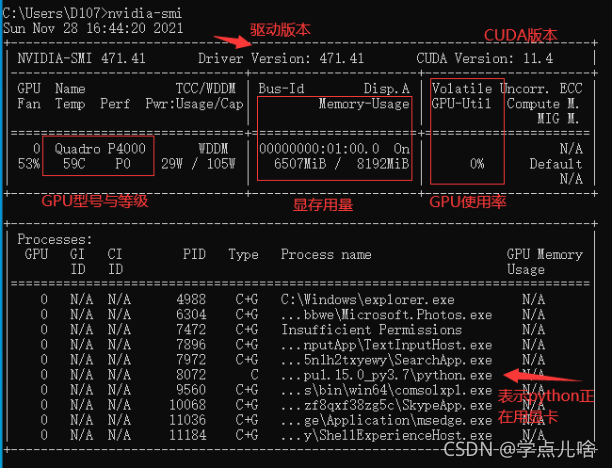# [Solved] Tensorflow-gpu Error: self._traceback = tf_stack.extract_stack()

Tensorflow GPU reports an error of self_ traceback = tf_ stack.extract_ stack()

Reason 1: the video memory is full

At this time, you can view the GPU running status by entering the command ` NVIDIA SMI` in CMD,
most likely because of the batch entered_ Size or the number of hidden layers is too large, and the display memory is full and the data cannot be loaded completely. At this time, the GPU will not start working (similar to memory and CPU), and the utilization rate is 0%Solution to reason 1:
1. turn down bath_Size and number of hidden layers, reduce the picture resolution, close other software that consumes video memory, and other methods that can reduce the occupation of video memory, and then try again. If the video memory has only two G’s, it’s better to run with CPU
2.
1. Use with code

``````os.environ['CUDA_VISIBLE_DEVICES'] = '/gpu:0'

config = tf.compat.v1.ConfigProto(allow_soft_placement=True)
config.gpu_options.per_process_gpu_memory_fraction = 0.7
tf.compat.v1.keras.backend.set_session(tf.compat.v1.Session(config=config))
``````

Reason 2. There are duplicate codes and the calling programs overlap
There are many reasons for the tensorflow error self_traceback = tf_stack.extract_stack()
the error codes are as follows:

``````import tensorflow as tf

a = tf.Variable(5., tf.float32)
b = tf.Variable(6., tf.float32)
num = 10
model_save_path = './model/'
model_name = 'model'
saver = tf.train.Saver()

with tf.Session() as sess:
init_op = tf.compat.v1.global_variables_initializer()
sess.run(init_op)
for step in np.arange(num):
saver.save(sess, os.path.join(model_save_path, model_name), global_step=step)
print("Parameters saved successfully!")
a = tf.Variable(5., tf.float32)
b = tf.Variable(6., tf.float32) # Note the repetition here
num = 10
model_save_path = './model/'
model_name = 'model'
saver = tf.train.Saver()    # Note the repetition here

with tf.Session() as sess:
init_op = tf.compat.v1.global_variables_initializer()
sess.run(init_op)
ckpt = tf.train.get_checkpoint_state(model_save_path)
if ckpt and ckpt.model_checkpoint_path:
saver.restore(sess, ckpt.model_checkpoint_path)
``````

Running the code will report an error: self_traceback = tf_stack.extract_stack()

Reason 2 solution
when ` Saver = TF.Train.Saver()` in parameter loading is commented out or commented out

``````a = tf.Variable(5., tf.float32)
b = tf.Variable(6., tf.float32) # Note the repetition here
``````

The model will no longer report errors. I don’t know the specific reason.